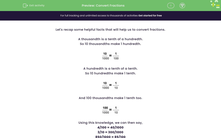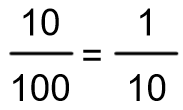# Equivalent Fractions

In this worksheet, students will be asked to convert fractions using either tenths, hundredths or thousandths as the denominator.Key stage:  KS 2

Curriculum topic:   Number: Fractions, Decimals and Percentages

Curriculum subtopic:   Convert Fractions

Popular topics:   Fractions worksheets, Equivalent Fractions worksheets

Difficulty level:#### Worksheet Overview

Let's recap some helpful facts that will help us to convert fractions.

A thousandth is a tenth of a hundredth.

So 10 thousandths make 1 hundredth.A hundredth is a tenth of a tenth.

So 10 hundredths make 1 tenth.And 100 thousandths make 1 tenth too.Using this knowledge, we can then say,

4/100 = 40/1000

3/10 = 300/1000

850/1000 = 85/100

To multiply by 10, we move the digits one column to the left.

To multiply by 100, we move the digits two columns to the left.

To multiply by 1000, we move the digits three columns to the left.

To divide by 10, 100 and 1000, we do the same steps as above, except the digits move to the right instead of left because the number gets smaller when we divide.

### What is EdPlace?

We're your National Curriculum aligned online education content provider helping each child succeed in English, maths and science from year 1 to GCSE. With an EdPlace account you’ll be able to track and measure progress, helping each child achieve their best. We build confidence and attainment by personalising each child’s learning at a level that suits them.

Get started